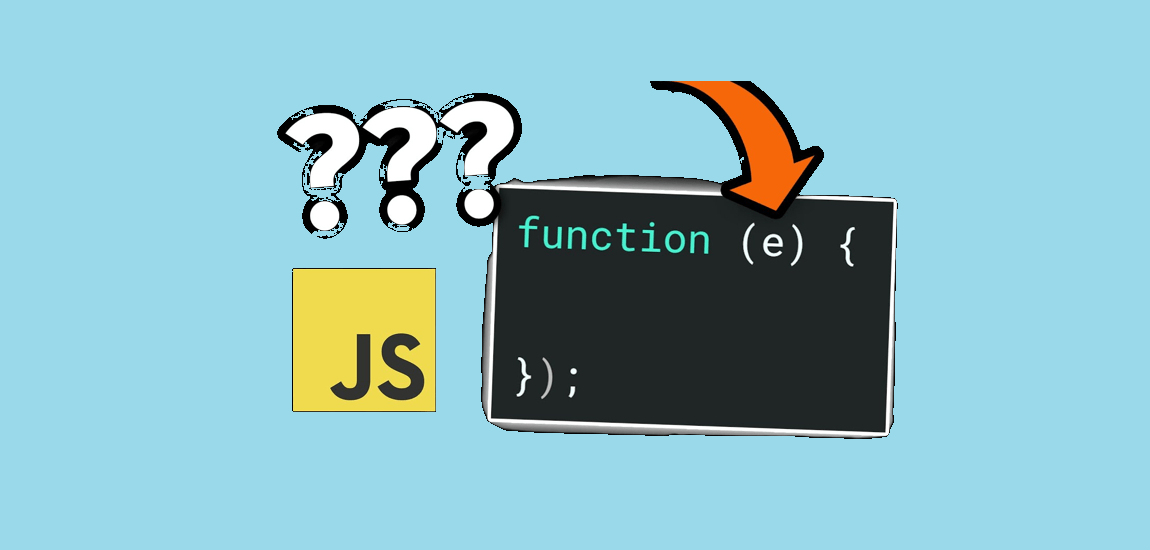# What is e in JavaScript? Understanding the Mathematical Constant

The letter “e” in JavaScript is a commonly used abbreviation that has several meanings depending on the context. In most cases, “e” refers to Euler’s number, a mathematical constant that is approximately equal to 2.71828. This number is used in various mathematical calculations, including logarithms and exponential functions.

In addition to representing Euler’s number, “e” can also be used as a variable name in JavaScript code. This variable can be assigned any value, including numbers, strings, and objects. However, it is generally recommended to avoid using single-letter variable names in order to improve code readability and prevent potential conflicts with other variables.

Overall, understanding the various meanings of “e” in JavaScript is essential for writing effective and efficient code. By keeping these different contexts in mind, developers can ensure that their code is accurate and easy to understand for both themselves and other programmers.## What is e in JavaScript?

JavaScript is a popular programming language that is used to create interactive and dynamic web pages. One of the essential concepts of JavaScript is the use of variables, and one such variable is “e.”

### Definition of e in JavaScript

In JavaScript, “e” is a mathematical constant that represents Euler’s number. Euler’s number is a mathematical constant that is approximately equal to 2.71828. It is named after the famous Swiss mathematician, Leonhard Euler, who discovered it in the 18th century.

In JavaScript, the constant “e” is stored as a property of the Math object. Therefore, it can be accessed using the Math.E property. The value of “e” is a predefined constant in JavaScript, which means that it cannot be changed or modified during the execution of a program.

### Why is e used in JavaScript?

The constant “e” is used in JavaScript to perform mathematical calculations and functions. For example, the Math.exp() function in JavaScript uses “e” to calculate the exponential value of a given number. Similarly, the Math.log() function uses “e” to calculate the natural logarithm of a given number.

Moreover, the constant “e” is used in JavaScript to perform complex mathematical calculations such as calculus, differential equations, and probability. It is also used in various scientific and financial applications that require precise mathematical calculations.

In conclusion, “e” is an essential mathematical constant in JavaScript that is used to perform various mathematical calculations and functions. Its value cannot be changed, and it is stored as a property of the Math object.

## How to Use e in JavaScript

The variable `e` is commonly used in JavaScript to refer to the event object that is passed as an argument to event handlers. It provides a lot of useful information about the event, such as the type of event, the target element, and the position of the mouse.

### Examples of Using e in JavaScript

Here are a few examples of how to use `e` in JavaScript:

• To prevent the default behavior of a link when it is clicked, you can use `e.preventDefault()`.
• To get the position of the mouse when it is clicked, you can use `e.clientX` and `e.clientY`.
• To get the target element of an event, you can use `e.target`.

``````
console.log(e); // 'e' is the event object, which contains information about the click event
});

``````

In this case, `e` is an object that contains information about the event that occurred, like which element was clicked, the coordinates of the click, the time the event was created, and much more.

Another instance where `e` might be encountered is in the context of mathematical constants or calculations, where `e` is the base of the natural logarithm, approximately equal to 2.718281828459.

``````
let exponential = Math.E; // this will give you the constant 'e' value

``````

It’s important to note that `e` does not have a specific meaning in JavaScript itself – it’s a convention developers adopt. Its meaning is defined by the context in which it’s used.

### Best Practices for Using e in JavaScript

When using `e` in JavaScript, it’s important to follow some best practices to ensure your code is clean, efficient, and easy to maintain. Here are a few tips:

• Always declare the `e` variable as the first argument of your event handler function. This clarifies that you are using the event object and makes your code more readable.
• Only access the properties and methods of `e` that you need. This can help improve your code’s performance by reducing unnecessary computations.
• Use descriptive variable names for the properties and methods of `e` that you are using. This makes your code more readable and easier to understand.

By following these best practices, you can use `e` in JavaScript to write clean, efficient, and maintainable code.